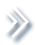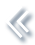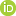####ArchivesReview Articles

## Statistical analysis of dependent competing risks model in constant stress accelerated life testing with progressive censoring based on copula function

Xuchao Bai ,

Department of Applied Mathematics, Northwestern Polytechnical University, Xi'an, Chinabaixuchao@126.com

Yimin Shi ,

Department of Applied Mathematics, Northwestern Polytechnical University, Xi'an, Chinaymshi@nwpu.edu.cn

Yiming Liu ,

Department of Applied Mathematics, Northwestern Polytechnical University, Xi'an, ChinaBin Liu

School of Applied Science, Taiyuan University of Science and Technology, Taiyuan, ChinaPages 48-57 | Received 16 Aug. 2017, Accepted 14 Apr. 2018, Published online: 01 May. 2018,
• Abstract
• References
• Citations

ABSTRACT

In this paper, we consider the statistical analysis for the dependent competing risks model in the constant stress accelerated life testing with Type-II progressive censoring. It is focused on two competing risks from Lomax distribution. The maximum likelihood estimators of the unknown parameters, the acceleration coefficients and the reliability of unit are obtained by using the Bivariate Pareto Copula function and the measure of dependence known as Kendall's tau. In addition, the 95% confidence intervals as well as the coverage percentages are obtained by using Bootstrap-p and Bootstrap-t method. Then, a simulation study is carried out by the Monte Carlo method for different measures of Kendall's tau and different testing schemes. Finally, a real competing risks data is analysed for illustrative purposes. The results indicate that using copula function to deal with the dependent competing risks problems is effective and feasible.

## References

1. Aristidis, K. N. (2013). On the estimation of normal copula discrete regression models using the continuous extension and simulated likelihood. Journal of Statistical Planning and Inference, 143, 19231937[Google Scholar]
2. Balakrishnan, N., & Han, D. (2008). Exact inference for a simple step-stress model with competing risks for failure from exponential distribution under Type-II censoring. Journal of Statistical Planning and Inference, 138, 41724186[Google Scholar]
3. Balakrishnan, N., & Lai, C. D. (2009). Continuous bivariate distributions. New York, NY: Springer Press[Google Scholar]
4. Beyersmann, J., Schumacher, M., & Allignol, A. (2012). Competing risks and multistate models with R. Springer[Google Scholar]
5. Bourguignon, M., Saulo, H., & Fernandez, R. N. (2016). A new Pareto-type distribution with applications in reliability and income data. Physica A, 457, 166175[Google Scholar]
6. Cheng, G., Zhou, L., Chen, X., & Zhuang, J. (2014). Efficient estimation of semiparametric copula models for bivariate survival data. Journal of Multivariate Analysis, 123, 330344[Google Scholar]
7. Cramer, E., & Schmiedt, A. B. (2011). Progressively Type-II censored competing risks data from Lomax distributions. Computational Statistics and Data Analysis, 55(3), 12851303[Google Scholar]
8. Dimitrova, D. S., Haberman, S., & Kaishev, V. K. (2013). Dependent competing risks: Cause elimination and its impact on survival. Insurance: Mathematics and Economics, 23, 464477[Google Scholar]
9. Dixit, U. J., & Nooghabi, M. J. (2010). Efficient estimation in the Pareto distribution. Statistical Methodology, 7, 687691[Google Scholar]
10. Fernández, A. J. (2014). Computing optimal confidence sets for Pareto models under progressive censoring. Journal of Computational and Applied Mathematics, 258, 168180[Google Scholar]
11. Grothe, O., & Hofert, M. (2015). Construction and sampling of Archimedean and nested Archimedean Levy copulas. Journal of Multivariate Analysis, 138, 182198[Google Scholar]
12. Han, D., & Balakrishnan, N. (2010). Inference for a simple step-stress model with competing risks for failure from the exponential distribution under time constraint. Computational Statistics and Data Analysis, 54, 20662081[Google Scholar]
13. Helu, A., Samawi, H., & Raqab, M. Z. (2015). Estimation on Lomax progressive censoring using the EM algorithm. Journal of Statistical Computation and Simulation, 85(5), 10351052[Taylor & Francis Online], [Google Scholar]
14. Jia, X., & Cui, L. (2012). Reliability research of k-out-of-n: G supply chain unit based on copula. Communications in Statistics - Theory and Methods, 41(21), 40234033[Taylor & Francis Online], [Google Scholar]
15. Jia, X., Wang, L., & Wei, C. (2014). Reliability research of dependent failure units using copula. Communications in Statistics - Simulation and Computation, 43(8), 18381851[Taylor & Francis Online], [Google Scholar]
16. Liu, F., & Shi, Y. (2017). Inference for a simple step-stress model with progressively censored competing risks data from Weibull distribution. Communications in Statistics - Theory and Methods, 46(14), 72387255[Taylor & Francis Online], [Google Scholar]
17. Mazucheli, J., & Achcar, J. A. (2011). The Lindley distribution applied to competing risks lifetime data. Computer Methods and Programs in Biomedicine, 104, 188192[Google Scholar]
18. Muliere, P., & Scarsini, M. (1987). Characterization of a Marshall-Olkin type class of distributions. Annals of Institute of Statistical Mathematics, 39, 429441[Google Scholar]
19. Nelsen, B. (2006). An introduction to copulas (2nd ed.). New York, NY: Springer Press[Google Scholar]
20. Pareto, V. (1987). Cours dćonomie Politique. Lausanne[Google Scholar]
21. Sarhan, A. M., & El-Gohary, A. I. (2003). Estimations of parameters in Pareto reliability model in the presence of masked data. Reliability Engineering and Unit Safety, 82, 7583[Google Scholar]
22. Sarhan, A. M., Hamilton, D. C., & Smith, B. (2010). Statistical analysis of competing risks models. Reliability Engineering and Unit Safety, 95, 953962[Google Scholar]
23. Wu, M., & Shi, Y. (2016). Bayes estimation and expected termination time for the competing risks model from Gompertz distribution under progressively hybrid censoring with binomial removals. Journal of Computational and Applied Mathematics, 300, 420431[Google Scholar]
24. Wu, M., Shi, Y., & Zhang, C. (2017). Statistical analysis of dependent competing risks model in accelerated life testing under progressively hybrid censoring using copula function. Communication in Statistics- Simulation and Computation, 46(5), 40044017[Taylor & Francis Online], [Google Scholar]
25. Xu, A., & Tang, Y. (2012). Statistical analysis of competing failure modes in accelerated life testing based on assumed copulas. Chinese Journal of Applied Probability and Statistics, 28, 5162[Google Scholar]
26. Xu, A., & Zhou, S. (2017). Bayesian analysis of series system with dependent causes of failure. Statistical Theory and Related Fields, 1(1), 128140[Taylor & Francis Online], [Google Scholar]
27. Yang, M., Wei, C., & Fan, Q. (2014). Parameter estimation for Lomax distribution under Type II censoring. Advanced Materials Research, 912-914, 16631668[Google Scholar]
28. Yi, W., & Wei, G. (2007). Study on the reliability of dependence-parts vote unit based on copula functions. Journal of Southwest China Normal University (Natural Science), 32(6), 5255[Google Scholar]
29. Zhang, X., Shang, J., Chen, X., Zhang, C., & Wang, Y. (2014). Statistical inference of accelerated life testing with dependent competing failures based on copula theory. IEEE Transaction on Reliability, 63(3), 764780[Google Scholar]
30. Zhang, C., Shi, Y., Bai, X., & Fu, Q. (2017). Inference for constant-stress accelerated life tests with dependent competing risks from bivariate Birnbaum–Saunders distribution based on adaptive progressively hybrid censoring. IEEE Transactions on Reliability, 66(1), 111122[Google Scholar]